# Important Questions For You!

Chapter 1: The Solid State

Q. 1. What is the formula of a compound in which the element Y forms hcp lattice and atoms of X occupy 2/3rd of tetrahedral voids? [1M]

Q. 2. Give an example each of a molecular solid and an ionic solid. [1M]

Q. 3. An element with density 2.8 gcm-3 forms a fcc unit cell with edge length 4 × l0-8 cm. Calculate the molar mass of the element. [2M]
(Given: NA=6.022 × 1023 mol-1)

Q. 4.                                                                                            [2M]

1. What type of non-stoichiometric point defect is responsible for the pink colour of LiCl?
2. What type of stoichiometric defect is shown by NaCl?

Q. 5. How will you distinguish between the following pairs of terms?      [2M]

1. Tetrahedral and octahedral voids
2. Crystal lattice and unit cell

Q. 6. Tungsten crystallizes in body centred cubic unit cell. If the edge of the unit cell is 315.5 pm, what is the radius of tungsten atom?      [3M]

Q. 7. Iron has a body centred cubic unit cell with a cell dimension of 286.65 pm, the density of iron is 7.874 gcm-3. Use this information to calculate Avogadro's number. (At. Mass of Fe = 55.845 μ).    [3M]

Q. 8. An element with density 10 gcm−3 forms a cubic unit cell with edge length of 3 × 10−8 cm. What is the nature of the cubic unit cell if the atomic mass of the element is 81 gmol−1?                       [3M]

Q. 9. (a) An element has a body centered cubic structure with a cell edge of 288 pm. The density of the elements is 7.2 g cm-3. Calculate the number of atoms present is 208 g of the element.          [3M]
(b) With example explain difference between crystalline and amorphous solids.     [2M]

Chapter 2: Solutions

Q. 1. State Henry’s law. What is the significance of KH? [1M]

Q. 2. Define reverse osmosis. Give one use of it. [1M]

Q. 3. State Henry’s law. Why do gases always tend to be less soluble in liquids as the temperature is raised? [2M]

Q. 4. State Raoult’s law for the solution containing volatile components. Write two differences between an ideal solution and a non-ideal solution.      [2M]

Q. 5. Calculate the amount of CaCl2 (molar mass = 111 g mol−1) which must be added to 500 g of water to lower its freezing point by 2 K, assuming CaCl2 is completely dissociated. (Kf for water = 1.86 K kg mol−1)?     [3M]

Q. 6. Calculate the boiling point of solution when 4g of MgSO4 (M= 120 g mol-1) was dissolved in 100 g of water, assuming MgSO4 undergoes complete ionization. (Kb for water = 0.52 K kgmol-1)?                 [3M]

Q, 7. [5M]

1. Difference between molarity and molality for a solution. How does a change in temperature influence their values?
2. Calculate the freezing point of an aqueous solution containing 10.50 g of MgBr2 in 200 g of water. (Molar mass of MgBr2 = 184 g)
(Kf for water = 1.86 K kg mol-1)

Q. 8.                                                                                                    [5M]

1. Define the terms osmosis and osmotic pressure. Is the osmotic pressure of a solution a colligative property? Explain.
2. Calculate the boiling point of a solution prepared by adding 15.00 g of NaCl to 250.0 g of water. (Kb for water = 0.512 K kg mol-1, Molar mass of NaCl = 58.44 g). [5M]

Q. 9.                                                                               [5M]

1. Define the following terms :
(i) Molarity
(ii) Molal elevation constant (Kb)
2. A solution containing 15 g urea (molar mass = 60 g mol-1) per litre of solution in water has the same osmotic pressure (Isotonic) as a solution of glucose (molar mass = 180 g mol-1) in water. Calculate the mass of glucose present in millitre of its solution.

Q. 10.                                                                               [5M]

1. What type of deviation is shown by a mixture of ethanol and acetone? Give reason.
2. A solution of glucose (molar mass = 180 g mol-1) in water is labelled as 10% (by mass). What would be the molality and molarity of the solution? (Density of solution= 1.2 g mL-1).

Chapter 3: Electrochemistry

Q. 1. When 5 V potential difference is applied across a wire of length 0.1 m, the drift speed of electrons is 2.5 × 10-4 m/s. If the electron density in the wire is 8 × 1028 m-3, calculate the resistivity of the material of the wire. [2M]

Q. 2. In a cell reaction, the equilibrium constant Kc is less than one. [2M]

1. Is EӨ for the cell positive or negative?
2. What will be the value of Kc if EӨcell = 0?

Q. 3. Calculate emf of the following cell at 25°C:     [3M]
Sn (s) | Sn2+ (0.001 M) || H+ (0.01 M) | H2 (g) (1 bar) | Pt (sq)Q. 4. Calculate the emf of the cell Zn|Zn2+ (0.1 M) || Cd2+ (0.01 M)|Cd at 298 K, [Given E°Zn2+/Zn = - 0.76 V and E°Cd2+/Cd = - 0.40 V ] [3M]

Q. 5.                                                                                      [5M]

1. Calculate the emf of the cell
Mg(s) |Mg2+ (0.1 M) Cu2+ (1 × 10-3 M)| Cu(s)
Given: E° Cu2+/Cu = +0.34 V, E° Mg2+/Mg = -2.37 V,
2. Explain with examples the terms weak and strong electrolytes.

Q. 6.                                                                                     [5M]

1. The resistance of a conductivity cell containing 0.001 M KCl solution at 298 K is 1500 Ω . What is the cell constant, if the conductivity of 0.001 M KCl solution at 298 K is 0.146 ×10−3 Scm-1?
2. What is meant by limiting molar conductivity?

Q. 7. Conductivity of 0.00241 M acetic acid solution is 7.896 × 10-5 S cm-1. Calculate its molar conductivity in this solution. Iffor acetic acid be 390.5 S cm2 mol-1, what would be its dissociation constant? [5M]

Q. 8. In a button cell, widely used in watches and other devices, the following reaction takes place:                    [5M]

Zn(s) + Ag2O(s) + H2O(I)  → Zn2+ (aq) + 2 Ag (s) + 2OH(aq)

1. Determine EΘcell and Δr G° for the reaction. Given

2. Explain with examples the terms weak and strong electrolytes. How can these be distinguished?

Chapter 4: Chemical Kinetics

Q. 1. A first order reaction has a rate constant of 0.0051 min-1. If we begin with 0.10 M concentration of the reactant, what concentration of the reactant will be left after 3 hours? [2M]

Q. 2. The rate constant for a reaction of zero order in A is 0.0030 molL−1s−1. How long will it take for the initial concentration of A to fall from 0.10 M to 0.075 M.                 [2M]

Q. 3. Nitrogen pentaoxide decomposes according to equation:

2N2O5(g) → 4NO2(g) + O2(g)

This first order reaction was allowed to proceed at 40 °C and the data below were collected:

 [N2O5] (M) Time (min) 0.400 0.00 0.289 20.0 0.209 40.0 0.151 60.0 0.109 80.0
2. What will be the concentration of N2O5 after 100 minutes?
3. Calculate the initial rate of reaction.

Q. 4. For the first order thermal decomposition reaction, the following data were obtained:                  [3M]Calculate the rate constant.
(Given: log 2 = 0.301, log3 = 0.4771, log 4 = 0.6021)

Q. 5.                                                                                                        [5M]

1. What is rate of reaction? Write two factors that affect the rate of reaction.
2. The decomposition of  N2O5, 2N2O5 (g) ⇄ 4NO2(g) + O2(g) is a first-order reaction. After 30 min from the start of decomposition in a closed vessel, the total pressure developed is found to be 284.5 mmHg. On complete decomposition, the total pressure is 584.5 mmHg. Calculate the rate constant of the reaction.

Q 6.                                                                                                   [5M]

1. For a reaction A + B ⟶ P, the rate is given by
Rate = k [A] [B]2
(i)    How is the rate of reaction affected if the concentration of B is doubled?
(ii)   What is the overall order of reaction if A is present in large excess?
2. A first order reaction takes 23.1 minutes for 50% completion. Calculate the time required for 75% completion of this reaction.(log 2 = 0.301, log 3 = 0.4771, log 4 = 0.6021).

Chapter 5: Surface Chemistry

Q 1. What is the effect of temperature on chemisorption?                   [1M]

Q 2. Define 'electrophoresis'?                                                           [1M]

Q 3. Write a method by which lyophobic colloids can be coagulated.     [1M]

Q 4. Explain how the phenomenon of adsorption finds applications in each of the following processes:  [3M]

1. Production of high vacuum
2. Controlling humidity
3. Froth floatation process

Q 5. Define each of the following terms:                   [3M]

1. Micelles
2. Peptization
3. Desorption?

Q 6. Define the following terms:                              [3M]

1. Lyophilic colloid
2. Zeta potential
3. Associated colloids

Chapter 6: The p-Block Elements

Q 1. Draw the structure of XeF2 molecule.      [1M]

Q 2. Pb(NO3)2 on heating gives a brown gas which undergoes dimerisation on cooling? Identify the gas.      [1M]

Q 3. Write the structures of the following:      [2M]

1. BrF3
2. XeF4

Q 4.                                                            [2M]
What happens when:

1. SO2 gas is passed through an aqueous solution Fe+3 salt?
2. XeF4 reacts with SbF5?

Q 5. Write the structures of the following species:       [2M]

1. H2S2O8
2. H2S2O7

Q 6. Complete the following chemical equations: (Any three)    [3M]

1. NaOH(aq) + Cl2(g)
(Hot and conc.)
2. XeF6(s) + H2O(l) →
3. 2NaOH + SO2
4. XeF4 + H2O →

Q 7. How would you account for the following?     [3M]

1. The value of electron gain enthalpy with negative sign for sulphur is higher than that for oxygen.
2. NF3 is an exothermic compound but NCl3 is endothermic compound.
3. ClF3 molecule has a T-shaped structure and not a trigonal planar one.

Q 8.    Explain the following observations giving appropriate reasons:      [3M]

1. The stability of +5 oxidation state decreases down the group in group 15 of the periodic table.
2. Fluorine exhibits only -1 oxidation state whereas other halogens exhibit +1, +3, +5 and +7 oxidation states also.
3. Halogens are strong oxidizing agents.

Q 9.                                                                   [5M]

1. Account for the following:
(i) Interhalogens are more reactive than pure halogens.
(ii) N2 is less reactive at room temperature.
(iii) Reducing character increases from NH3 to BiH3.
2. Draw the structures of the following:
(i)    HOClO3
(ii)    XeF4

Q 10.                                                                 [5M]

1. How can you prepare Cl2 from HCl and HCl from Cl2. Write reactions only.
2. Which noble gas has the lowest boiling point?
3. Fluorine is a stronger oxidising agent than chlorine. Why?
4. How are XeO3 and XeOF4 are prepared?
5. Complete the equation:
PbS + O3

Chapter 7: d- and f-Block Elements

Q 1. Assign reasons for the following:                                        [2M]

1. Copper (I) ion is not known in aqueous solution.
2. Silver atom has completely filled d orbitals in its ground state but it is a transition element.

Q 2. Explain the following observations:                                    [2M]

1. Generally there is an increase in density of elements from titanium (Z = 22) to copper (Z = 29) in the first series of transition elements.
2. Transition elements and their compounds are generally found to be good catalysts in chemical reactions.

Q 3.                                                                                      [3M]

1. Name the method used for the refining of zirconium.
2. What is the role of CO in the extraction of Iron?
3. Reduction of metal oxide to metal becomes easier if the metal obtained is in liquid state. Why?

Q 4. What is meant by ‘disproportionation’? Give two examples of disproportionation reaction in aqueous medium. [3M]

Q 5.                                                                                       [5M]

1. Account for the following:
(i) The d1 configuration is very unstable in ions.
(ii) Cr+2 is a strong reducing agent.
(iii) Cu+2 salts are coloured, while Zn+2 salts are white.
2. Name an important alloy which contains some of the lanthanoid metals. Mention its uses.

Q 6. The elements of 3d transition series are given as:             [5M]
Sc Ti V Cr Mn Fe Co

1. Write the element which shows maximum number of oxidation states. Give reason.
2. Which element has the highest m.p.?
3. Which element shows only +3 oxidation state?
4. Which element is a strong oxidising agent in +3 oxidation state and why?

Q 7. Assign reasons for the following:                                       [5M]

1. The enthalpies of atomisation of transition elements are high.
2. The transition metals and many of their compounds act as good catalyst.
3. From element to element, the actinoid contraction is greater than the lanthanoid contraction.
4. The Eo value for the Mn3+/Mn2+ couple is much more positive than that for Cr3+/Cr2+.
5. Scandium (Z = 21) does not exhibit variable oxidation states and yet it is regarded as a transition element.

Q 8.                                                                                      [5M]

1. What may be the possible oxidation state of the transition metals with the following d electronic configurations in the ground state of their atoms: 3d34s2, 3d54s2 and 3d64s2. Indicate relative stability of oxidation states in each case.
2. Write steps involved in the preparation of (i) Na2CrO4 from chromite ore and (ii) K2MnO4 from pyrolusite ore.

Chapter 8: Coordination of Compounds

Q 1.                                                                                       [2M]

1. Write down the IUPAC name of the following complex:
[Co(NH3)5 (NO2)](NO3)2
2. Write the formula for the following complex:
Potassium tetracyanidonickelate (II)

Q 2. Name the following coordination compounds according to IUPAC system of nomenclature: [2M]

1. [Co(NH3)4(H2O)Cl]Cl2
2. [CrCl2(en)2]Cl, (en=ethane - 1,2-diamine)

Q 3. When a coordination compound CoCl3.6NH3 is mixed with AgNO3, 3moles of AgCl are precipitated per mole of the compound. Write                                                                           [2M]

1. Structural formula of the complex
2. IUPAC name of the complex

Q 4.                                                                                            [3M]

1. For the complex [Fe(H2O)6]+3, write the hybridisation, magnetic character and spin of the complex. (At, number : Fe = 26)
2. Draw one of the geometrical isomers of the complex [Pt (en)2Cl2]+2 which is optically inactive.

Q 5.                                                                                           [3M]

1. Draw the geometrical isomers of complex [Co(en)2Cl2]+.
2. On the basis of crystal field theory, write the electronic configuration for d4 ion if ∆0 > P.
3. [NiCl4]2− is paramagnetic, while [Ni(CO)4] is diamagnetic, though both are tetrahedral. Why? (Atomic number of Ni = 28)

Q 6.                                                                                              [5M]

1. What are ambidentate ligands? Give an example.
2. Write the names of the following:
(i) K3[Cr(C2O4)3]
(ii) Pt[(NH3)2Cl2]
3. Draw the structure of the cis isomer of [Co(NH3)4Cl2]+.

Chapters 9: Haloalkanes and Haloarenes

Q 1. Which would undergo SN1 reaction faster in the following pair and why? [1M]Q 2. Write the structure of an isomer of compound C4H9Br which is most reactive towards SN1 reaction. [1M]

Q 3. Write the IUPAC name of the following compound: [1M]

(CH3)3CCH2Br

Q 4. Identify the chiral molecule in the following pair: [1M]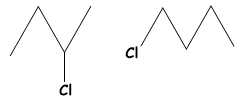Q 5. Answer the following:                                       [3M]

1. Haloalkanes easily dissolve in organic solvents, why?
2. What is known as a racemic mixture? Give an example.
3. Of the two bromo derivatives, C6H5CH(CH3)Br and C6H5CH(C6H5)Br, which one is more reactive in SN1 substitution reaction and why?

Q 6. How do you convert the following:                    [3M]

1. Prop-1-ene to 1-fluoropropane
2. Chlorobenzene to 2-chlorotoluene
3. Ethanol to propanenitrile

Q 7. Write the main products when                           [3M]

1. n-butyl chloride is treated with alcoholic KOH.
2. 2, 4, 6-trinitrochlorobenzene is subjected to hydrolysis.
3. methyl chloride is treated with AgCN.

Q 8. How do you convert:                                        [3M]

1. Chlorobenzene to biphenyl
2. Propene to 1-iodopropane
3. 2-bromobutane to but-2-ene

Q 9. Write the major products(s) in the following:      [3M]

1.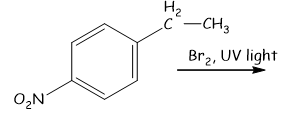2.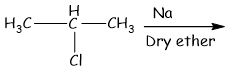3.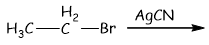Chapters 10: Alcohols, Phenols and Ethers

Q 1. Write the IUPAC name of the given compound:                             [1M]Q 2. How would you account for the following:                                    [2M]

1. Phenols are much more acidic than alcohols.
2. The boiling points of ethers are much lower than those of the alcohols of comparable molar masses.

Q 3. Give reasons for the following:                                                  [3M]

1. o-nitrophenol is more acidic than o-methoxyphenol.
2. Butan-1-ol has a higher boiling point than diethyl ether.
3. (CH3)3C–O–CH3 on reaction with HI gives (CH3)3C–I and CH3–OH as the main products and not (CH3)3C–OH and CH3–I.

Q 4. Write the final product(s) in each of the following reactions: [3M]

1.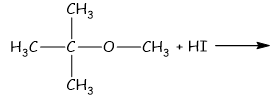2.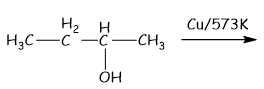3.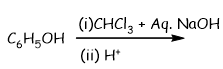Chapter 11: Aldehydes, Ketones and Carboxylic acid

Q 1. How would you account for the following:                                   [3M]

1. Aldehydes are more reactive than ketones towards nucleophiles.
2. The boiling points of aldehydes and ketones are lower than of the corresponding acids.
3. The aldehydes and ketones undergo a number of addition reactions.

Q 2. Give chemical tests to distinguish between:                                [2M]
1. Acetaldehyde and benzaldehyde
2. Propanone and propanol

Q 3.                                                                                               [5M]

(a) Write the products formed when CH3CHO reacts with the following reagents:

1. HCN
2. H2N - OH
3. CH3CHO in the presence of dilute NaOH

(b) Give simple chemical tests to distinguish between the following pairs of compounds:

1. Benzoic acid and Phenol
2. Propanal and Propanone

Q 4.                                                                                              [5M]

(a) Account for the following:

1. Cl - CH2COOH is a stronger acid than CH3COOH.
2. Carboxylic acids do not give reactions of carbonyl group.

(b) Out of CH3CH2-CO - CH2 - CH3 and CH3CH2- CH2- CO – CH3 which gives iodoform test.

(c) Write the chemical equations to illustrate the following name reactions:

1. Rosenmund reduction
2. Cannizzaro's reaction

Q 5.

1. Write the structural formula of 1 - phenylpentan - 1 - one.      [1M]
2. Write the structure of p-methylbenzaldehyde.                        [1M]
3. Draw the structure of 3-methylbutanal.                                 [1M]

Q 6. Write the IUPAC name of the compound:                                   [1M]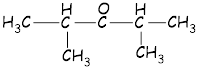Q 7. Predict the products of the following reactions:                          [3M]

1.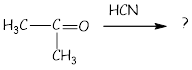2.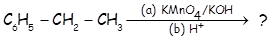3.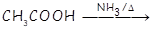Q 8.
1. Describe the following giving suitable examples:            [3M]
(i) Cannizzaro reaction
(ii) Aldol condensation
2. Give a chemical test to distinguish between ethanal and propanal.     [2M]
Q 9.                                                                                        [5M]
1. Write chemical equations of illustrate the following name bearing reactions:
(i) Cannizzaro's reaction
(ii) Hell- Volhard - Zelinsky reaction
2. Give chemical tests to distinguish between the following pairs of compounds:
(i) Propanal and Propanone
(ii) Acetophenone and Benzophenone
(iii) Phenol and Benzoic acid

Chapter 12: Amines

Q 1. Arrange the following compounds in an increasing order of their solubility in water:    [1M]

C6H5NH2, (C2H5)2NH, C2H5NH2
Give the IUPAC name of H2N - CH2 - CH2 - CH = CH2                                                   [1M]

Q 2. Write the chemical equations involved in the following reactions:                           [2M]

2. Carbylamine reaction

Q 3. Define the following terms in relation to proteins:                                                [2M]

2. Denaturation

Q 4. Account for the following:                                                                              [3M]

1. Electrophilic substitution in case of aromatic amines takes place more readily than benzene.
2. CH3CONH2 is a weaker base than CH3CH2NH2.
3. Nitrocompounds have higher boiling points than hydrocarbons having almost same molecular mass.

Q 5.                                                                                                                 [3M]

1. Explain why an alkylamine is more basic than ammonia.
2. How would you convert:
(i) Aniline to nitrobenzene
(ii) Aniline to iodobenzene?

Q 6. Account for the following observations:                                                         [3M]
1. pkb for aniline is more than that for methylamine.
2. Methylamine solution in water reacts with ferric chloride solution to give a precipitate of ferric hydroxide.
3. Aniline does not undergo Friedel-Crafts reaction.

Chapter 13: Biomolecules

Q 1. What are reducing sugars?                                           [1M]

Q 2. Describe what you understand by primary structure and secondary structure of proteins.  [2M]

Q 3. List the reactions of glucose which cannot be explained by its open chain structure.         [2M]

Q 4. What is essentially the difference between -form of glucose and -form of glucose? Explain.   [2M]

Q 5.                                                                                                                        [3M]

1. What are the common types of secondary structure of proteins?
2. What is the difference between native protein and denatured protein?
3. Write the name of the vitamin responsible for the coagulation of blood.

Q 6.                                                                                            [3M]

1. What are the different types of RNA found in the cell?
2. What type of linkage is present in nucleic acids?
3. Give one example each for fibrous protein and globular protein.

Q 7.                                                                             [5M]

1. State two main differences between globular proteins and fibrous proteins.
2. Based on their chemical composition, state how are lipids classified? Give one example of each class.

Q 8.                                                                               [5M]
1. The two strands in DNA are not identical but are complementary. Explain.
2. Write the important structural and functional differences between DNA and RNA.

Case Study Questions

Q 1. Every generation of each and every species resembles its ancestors in many ways. It has been observed that the nucleus of a living cell is responsible for this transmission of inherent characters, also called heredity. The particles in the nucleus of the cell, responsible for heredity, are called chromosomes which are made of proteins and another type of biomolecules called nucleic acids. These are mainly of two types DNA and RNA. Since nucleic acids are long chain polymers of nucleotides, they are also called polynucleotides. James Watson and Francis Crick gave a double helix structure for DNA.α

1.  Which sugars are present in DNA and RNA?
a) β-D-2-deoxyribose, β-D-ribose
b) β-D-2-deoxyribose, β-L-ribose
c) α-D-2-deoxyribose, β-D-ribose
d) α-D-2-deoxyribose, α-D-ribose

2. What are the different types of RNA?
a) r-RNA, t-RNA, g-RNA
b) m-RNA, r-RNA, a-RNA
c) t-RNA, m-RNA, r-RNA
d) t-RNA, n-RNA, m-RNA

3. What type of bond is present to keep DNA strands complementary to each other?
a) Covalent bond
b) Hydrogen bond
c) Metallic bond
d) Ionic bond

4. What are the bases present in DNA?
a) A, G, C, T
b) A, T, C, U
c) D, T, C, G
d) A, T, C, G

Q 2. Leclanche cell is a primary cell. It consists of a cylindrical zinc container which acts as the anode. A graphite rod surrounded by a paste of manganese dioxide acts as the cathode. These are in contact with a thick paste of ammonium chloride and zinc chloride which acts as the electrolyte.

1. What is the reaction taking place at the anode?
a) Zn(s) → Zn+2 + 2e-
b) Zn+2 → Zn(s) +2e-
c) MnO2 ++ e→ MnO(OH) + NH3
d) None of these

2. How much electricity in terms of faraday is produced by the oxidation of one mole of Zn?
a) 3F
b) 2F
c) 1F
d) 4F

3. Leclanche cell is considered as the primary cell because
a) Reaction inside the cell is not reversed.
b) Reaction inside the cell is reversed.
c) It is used in transitor.
d) None of these

4. Lelanche cell is not use in
a) Tape recorder
c) Flashlight
d) None of these.

Q 3. Potassium permanganate is manufactured on a large scale by the alkaline oxidative fusion of pyrolusite ore followed by electrolytic oxidation in alkaline medium. It is crystalline, deep purple in colour and acts as an oxidizing agent in the neutral, alkaline and acidic media. In acidic medium, it is used in volumetric analysis for estimation of ferrous salts, oxalates, etc. The titrations are carried out in presence of H2SO4. For use an oxidizing agent, KMnO4 should be stored in dark bottles and standardized just before use with standard oxalic acid solution or Mohr salt solution. In an experiment on titration, 13.4 g of dry pure sodium oxalate (molar mass=134 g mol-1) was dissolved in 100 mL of distilled water and then 100 mL of 2M H2SO4 were added. The solution was cooled to 25-30oC. Now to this solution, 0.1 M KMnO4 solution was added till a very faint pink colour persisted.
In these questions (Q. No 5-8 , a statement of assertion followed by a statement of reason is given. Choose the correct answer out of the following choices).
a) Assertion and reason both are correct statements and reason is correct explanation for assertion.
b) Assertion and reason both are correct statements but reason is not correct explanation for assertion.
c) Assertion is correct statement but reason is wrong statement.
d) Assertion is wrong statement but reason is correct statement.

1. Assertion: When pyrolusite is fused with KOH and KNO3, we get KMnO4.
Reason: MnO2 is pyrolusite.
2. Assertion: KMnO4 is purple in colour.
Reason: In KMnO4, an electron is momentarily transferred from O to metal.
3. Assertion: Mohr salt is preferred over FeSO4.7H2O for standardization of KMnO4 solution.
Reason: FeSO4.7H2O contains only ferrous ions whereas ferrous sulphate contains some Ferric ions.
4. Assertion: If instead of H2SO4, HCl or HNO3 of suitable concentration were used, the volume of KMnO4 solution used would have been more than HCl.
Reason: If instead of H2SO4, HCl or HNO3 of suitable concentration were used, the volume of KMnO4 solution used would have been more than HNO3.

Q 4. The pressure exerted by the vapour when in equilibrium with a liquid or a solution at a particular temperature is known as vapour pressure. When two (or more) components of a liquid solution evaporate, the vapour contain molecules of each substance. All liquid solutions of non-volatile solutes (solutes that have no tendency to evaporate) have lower vapour pressures than their pure solvents. The vapour pressure of such a solution is proportional to how much of the solution actually consists of the solvent. The proportionality is given by Raoult’s law (also known as the vapour-pressure concentration law) which says that the vapour pressure of the solution equals the mole fraction of the solvent multiplied by its vapour pressure when pure. In a binary solution of components A and B, if the interactive force between A—A, B---B and A—B are same the solution is ideal. Practically, no solution is ideal. Practically, no solution is ideal. A non-ideal solution is that solution in which solute and solvent molecules interact with one another with a different force than the forces of interaction between the molecules of pure components. If for the two components A and B, the force of interaction between the A-A and B-B, the non-ideal solutions have positive deviations. On the other hand, if the forces of interaction between A and B molecules are more than those between A—A and B---B, the non-ideal solutions have negative deviation.

The following questions are multiple choice questions. Choose the most appropriate answer:

1. If two liquids A and B form an ideal solution, then
a) The Gibbs energy of mixing is zero.
b) The entropy of mixing is zero.
c) The enthalpy of mixing is zero.
d) None of these

2. Vapour pressure of dilute aqueous solution of glucose is 750 mm of Hg at 373K. the mole fraction of the solute is:
a) 1/75
b) 75/76
c) 1/76
d) 1/10

3. Which of the following statements is correct for non-ideal solutions?
a) For solutions showing positive deviation, ΔHmix is positive.
b) For solutions, showing negative deviations, the interactions between the components are greater than pure components.
c) For solutions showing negative deviations, ΔVmix is positive but ΔHmix is negative.
d) For solutions showing negative deviations, ΔVmix and ΔHmix are positive.

4. The vapour pressure of a pure liquid A is 30mm of Hg at 300 K. The vapour pressure of this liquid in solution with liquid B is 24 mm of Hg. The mole fraction of A in solution obeying Raoult’s law is
a) 0.4
b) 0.3
c) 0.5
d) 0.8

Q 5. X-ray studies show that the packing of atoms in a crystal of a metal is found to be in layers such that starting from any layer, every fourth layer is exactly identical. The density of the metal is found to be 19.4 g cm-3 and its atomic mass is 197 u.

In these questions (a statement of assertion followed by a statement of reason is given. Choose the correct answer out of the followings).
a) Both assertion and reason are correct, and the reason is the correct explanation of the assertion.
b) Both assertion and reason are correct, but the reason is not the correct explanation of the assertion.
c) Assertion is correct, but reason is wrong.
d) Assertion is wrong, but reason is correct.

1. Assertion: The Coordination number of metal atom in the crystal is 12.
Reason: Metal has fcc arrangement.
2. Assertion: The fraction occupied by metal atoms in the crystal is 0.74.
Reason: Packing efficiency in fcc arrangement is 74%
3. Assertion: Assuming the metal atom to be spherical, its radius will be 103.5 pm.
Reason: For fcc, r =4. Assertion: The approximate number of unit cells present in 1 g of metal is 1.53 × 1021.
Reason: For fcc arrangement, there are 4 atoms per unit cell.

Q 6. Previously the term “colloids” was used for a category of substances, however, later the term colloidal state of matter was preferred. Colloidal dispersions have been classified into different types depending upon the physical state of the dispersed phase and the dispersed medium or the nature of interactions between them or the nature of the colloidal particles. They are prepared in the industry or in the laboratory by a number of methods and then purified. Their properties have also been studied in detail. Hardy and Schulze made a substantial contribution in studying the coagulation of the colloids. The protective action of lyophilic colloids was studied by Zsigmondy and he introduced a term, called “gold number”.

1. Lyophillic sols are more stable than lyophobic sols because
a) the colloidal particles have positive change
b) the colloidal particles have negative change
c) the colloidal particles are solvated
d) there are strong electrostatic repulsions between the particles

2. Which one of the following forms micelles in aqueous solution above certain concentration?
a) Dodecyl trimethyl ammonium chloride
b) Glucose
c) Urea
d) Pyridinium chloride

3. Identify the correct statement about colloids
a) Colloidal sulfur is a substance.
b) A colloid can be defined as homogenous system.
c) A colloidal state is intermediate between a true solution and a suspension
d) None of these.

4. Gold number is associated with
a) Electrophoresis
b) Amount of pure gold
c) Protective colloid
d) Precipitation of colloid

Q 7. The noble gases have closed-shell electronic configuration and are monoatomic gases under normal conditions. The low boiling points of the lighter noble gases are due to weak dispersion forces between the atoms and the absence of other interatomic interactions. The direct reaction of xenon with fluorine leads to a series of compounds with oxidation numbers +2, +4 and +6. XeF4 reacts violently with water to given XeO3. The compounds of Xenon exhibit rich stereochemistry and their geometries can be deduced considering the total number of electron pairs in the valence shell.

In these questions (a statement of assertion followed by a statement of reason is given. Choose the correct answer out of the followings).
a) Both assertion and reason are correct, and the reason is the correct explanation of the assertion.
b) Both assertion and reason are correct, but the reason is not the correct explanation of the assertion.
c) Assertion is correct, but reason is wrong.
d) Assertion is wrong, but reason is correct.

1. Assertion: The structure of XeOF4 is planar.
Reason: XeOF4 is octahedral with one position unoccupied.
2. Assertion: XeF2 oxidizes Cl- to Cl2.
Reason: XeF2 is oxidizing agent.
3. Assertion: Argon is used in metallurgical process.
Reason: Argon is having low reactivity with metal.
4. Assertion: XeF4 reduces I2 to I-.
Reason: XeF4 is oxidizing agent.

Q 8. The number of reacting species (atoms, ions or molecules) taking part in an elementary reaction which must collide simultaneously to bring about a chemical reaction is called molecularity of a reaction.
Order of a reaction is an experimental quantity; it can be zero and even a fraction but molecularity cannot be zero or a non-integer. The sum of powers of the concentration of the reactants in the rate law expression is called the order of that chemical reaction. The overall rate of the reaction is controlled by the slowest step in a reaction called the rate-determining step. So, in a complex reaction, molecularity of the slowest step is the same as the order of the reaction step in a reaction called the rate-determining step. So, in a complex reaction, molecularity of the slowest step is the same as the order of the reaction.
For a general reaction,

aA + bB → cC + dD

Rate = k[A]x[B]y
x+y = Order of reaction
k= Rate constant

1. What is the molecularity of the reaction
a) 1
b) 2
c) 0
d) 3

2. Find the order of the given reaction.
a) 1
b) 2
c) 3
d) 4

3. For the second-order reaction A + B → Product, the rate law is given by Rate = k[A]2 [B]x. What is the value of x?
a) 0
b) 1
c) 2
d) 3

4. For the zero-order reaction , if the concentration of A becomes double, then what will be the change in the rate of reaction?
a) increased
b) decreases
c) no change
d) can’t say anything

Q 9. The aggregation of Fe(OH)3 sol can be done by adding ionic solution, especially if the solution contains multiple charged atoms (e.g., phosphate ions). Coagulation is the process by which the dispersed phase of colloid undergoes aggregation, and thus separates from the continuous phase. In these questions (Q. No 5-8, a statement of assertion followed by a statement of reason is given. Choose the correct answer out of the following choices).
a) Assertion and reason both are correct statements and reason is correct explanation for assertion.
b) Assertion and reason both are correct statements but reason is not correct explanation for assertion.
c) Assertion is correct statement but reason is wrong statement.
d) Assertion is wrong statement but reason is correct statement.

1. Assertion: Fe(OH)3 is positively charged colloid.
Reason: Fe(OH)3 can be coagulated by negatively charged ions.
2. Assertion: KCl is more effective than MgCl2 in coagulating sulphur.
Reason: Greater the valence of the active ion or flocculating ion, greater will be its coagulating power.
3. Assertion: Arsenious sulphide is positively charged.
Reason: Aluminium hydroxide solution is negatively charged.
4. Assertion: Colloidal sulphur particles are negatively charged.
Reason: Colloidal sulphate particles are negatively charged.

Assertion-Reason Questions

In the following questions questions, a statement of assertion is followed by a statement of reason is given. Choose the correct answer out of the following choices.
a) Both assertion and reason are correct, and the reason is the correct explanation of the assertion.
b) Both assertion and reason are correct, but the reason is not the correct explanation of the assertion.
c) Assertion is correct, but reason is wrong.
d) Assertion is wrong, but reason is correct.

1. Assertion: Bromobenzene upon reaction with Br2/Fe gives 1, 4-dibromobenzene as the major product.
Reason: In bromobenzene, the inductive effect of the bromo group is more dominant than the mesomeric effect in directing the incoming electrophile.
2. Assertion: Iodine is sparingly soluble in water but fairly soluble in KI.
Reason: Iodine is non-polar.
3. Assertion: Aqueous gold colloidal solution is red.
Reason: The colour arises due to scattering of light by colloidal gold particles.
4. Assertion: Molecular mass of benzoic acid when determined by colligative properties is found to be high.
Reason: Dimerisation of benzoic acid.
5. Assertion: Speed of ions depends on the nature of ions only.
Reason: Mobility of ions depends on mass, charge and size of ions only.
6. Assertion: Decreasing concentration by dilution increases the equivalence conductance of aqueous NaCl solution.
Reason: With dilution, degree of ionisation of NaCl increases.
7. Assertion: MgCO3 is soluble in water when a current of CO2 is passed.
Reason: The solubility of MgCO3 is due to the formation of Mg(HCO3)2.
8. Assertion: A pink-coloured solution of potassium permanganate turns green on passing O3 through it.
Reason: K2MnO4 is oxidised by O3 to KMnO4.
9. Assertion: Ozonolysis of 3-hexyne followed by hydrolysis gives propanoic acid.
Reason: The byproduct H2O2 oxidises ozonide to acid.
10. Assertion: Benzonitrile is prepared by the reaction of chlorobenzene with potassium cyanide.
Reason: Cyanide (CN⎺ ) is a strong nucleophile.
11. Assertion: [Fe(H2O)5NO]SO4 is paramagnetic.
Reason: Fe in [Fe(H2O)5NO]SO4 has three unpaired electrons.
12. Assertion: In a strongly acidic solution, aniline becomes more reactive towards electrophilic reagents.
Reason: The amino group being completely protonated in strongly acidic solution, the lone pair of electrons on nitrogen is no longer available for resonance.
13. Assertion: Micelles are formed by surfactant molecules above the critical micelle concentration (CMC).
Reason: Conductivity of a solution having surfactant molecules decreases sharply at CMC.
14. Assertion: Heat absorbed during the isothermal expansion of an ideal gas against vacuum is zero.
Reason: Volume occupied by the molecules of an ideal gas is zero.
15. Assertion: Acetic acid does not undergo haloform reaction.
Reason: Acetic acid has no alpha hydrogen.
16. Assertion: The two strands in the double strand helix structure of DNA are complementary to each other
Reason: Disulphide bonds are formed between specific pairs of bases.
17. Assertion: Between SiCl4 and CCl4, only SiCl4 reacts with water.
Reason: SiCl4 is ionic and CCl4 is covalent.
18. Assertion: Geometrical isomers of the complex [M(NH3)4Cl2] are optically inactive.
Reason: Both geometrical isomers of the complex [M(NH3)4Cl2] possess an axis of symmetry.
19. Assertion: Aldol condensation can be catalysed by both acids and bases.
Reason: β-hydroxy aldehydes or ketones readily undergo acid-catalysed dehydration.
20. Assertion: NF3 is a weaker ligand than N(CH3)3.
Reason: NF3 ionises to give F ions in aqueous solution.
21. Assertion: In strongly acidic solution, aniline becomes more reactive towards electrophilic reagents.
Reason: The amino group being completely protonated in strongly acidic solution, the lone pair of electrons on nitrogen is no longer available for resonance.
22. Assertion: C2H5Br reacts with alcoholic solution of AgNO2 to form nitroethane as the major product.
Reason:is an ambident ion.
23. Assertion: The instantaneous rate of reaction can be studied in chemical kinetics.
Reason: Ionic reactions occur instantaneously.
24. Assertion: Styrene on reaction with HBr gives 1-bromo-1-phenylethane.